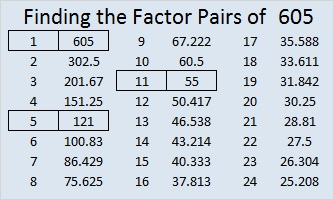# 605 and Level 4

605 is the hypotenuse of the Pythagorean triple 363-484-605. The greatest common factor of those three numbers should be fairly easy to spot.

6 – 0 + 5 = 11, obviously a multiple of 11, so 605 passes 11’s divisibility test and can be evenly divided by 11.

Coincidentally, OEIS.org shared some trivia about 605’s digits: 6 + 0 + 5 = 11, the greatest prime factor of 605.Print the puzzles or type the solution on this excel file: 10 Factors 2015-09-01

—————————————————————————————————

• 605 is a composite number.
• Prime factorization: 605 = 5 x 11 x 11, which can be written 605 = 5 x (11^2)
• The exponents in the prime factorization are 1 and 2. Adding one to each and multiplying we get (1 + 1)(2 + 1) = 2 x 3  = 6. Therefore 605 has exactly 6 factors.
• Factors of 605: 1, 5, 11, 55, 121, 605
• Factor pairs: 605 = 1 x 605, 5 x 121, or 11 x 55
• Taking the factor pair with the largest square number factor, we get √605 = (√121)(√5) = 11√5 ≈ 24.5967—————————————————————————————————This site uses Akismet to reduce spam. Learn how your comment data is processed.